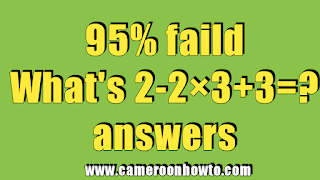# What's 2-2× 3+3 = ? Quiz Answer

0What's 2-2× 3+3 = ? Quiz Answer
What's 2-2× 3+3 = ? Quiz Answer

This math Quizzes is confusing many people on social media especially on Facebook. When we first published it,many people failed, many people had One and 9 as their answers.

What's 2-2× 3+3 = ? Math quiz and looks simple at a glance but because of some little anxiety, many people failed it.

Today I will solve in detail and give you the right answer. Also read: 10-10×10+10 = ? Trending math quiz answer

## What's 2-2× 3+3 = ?

The correct answer to "What's 2-2× 3+3 = ?" would be -1 not 9,this is because I am going to apply BODMAS in the solving. Get details below.

Be sure to share with friends, family and loved ones to test them.

In mathematics, BODMAS is an acronym for:

Bracket

Order of Operations

Division

Multiplication

Subtraction

This BODMAS is used to answer basic Mathematics quizzes and questions like the one above. Let's solve using BODMAS.

2 - 2 × 3+3 = ?

Since there's no Bracket, I will go straight to multiplication.

-2×3 = -6

So,2-6+ 3 =?

5-6 = ?

-1 =?

Therefore, the correct answer is -1

Conclusion

To pass these types of Quizzes, you need to apply BODMAS carefully else you will always get it wrongly.